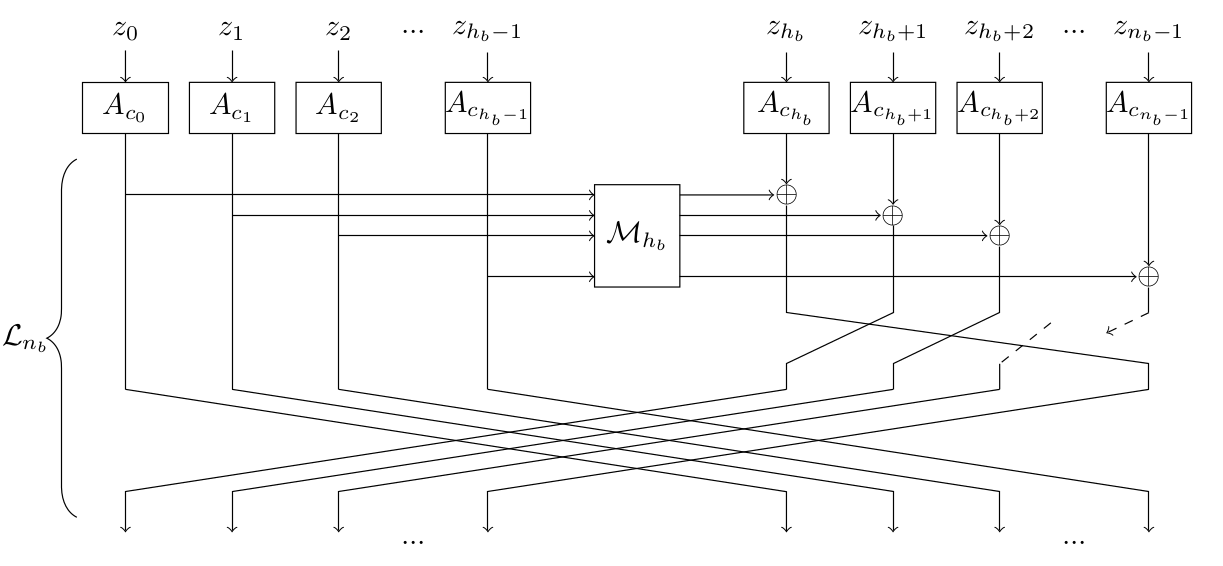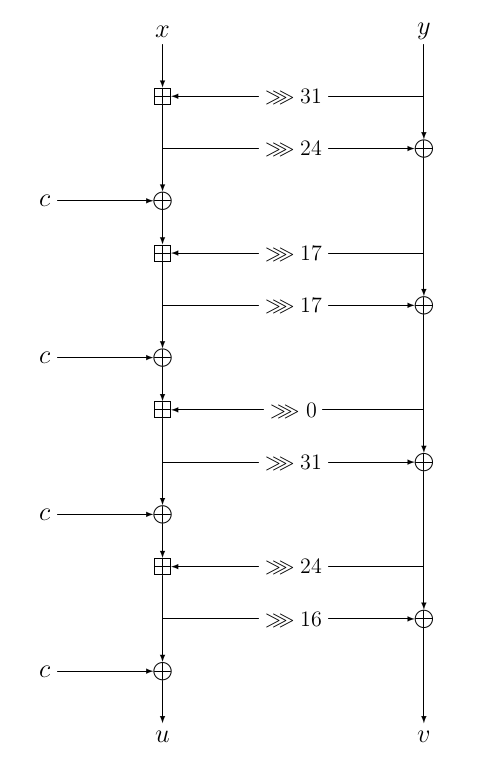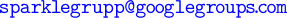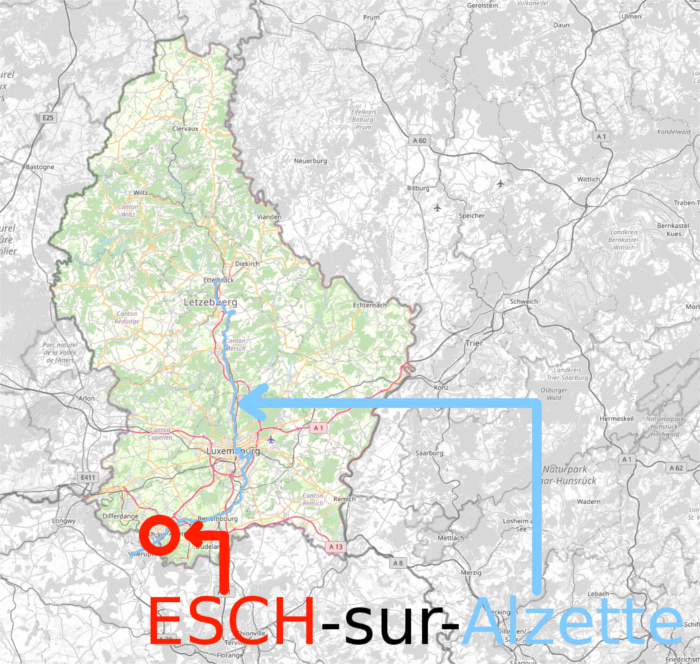# Sparkle Suite

## A collection of lightweight symmetric cryptographic primitives, finalist of the ongoing NIST lightweight standardisation effort.

The Sparkle-suite is a collection of lightweight symmetric cryptographic algorithms currently in the final round of the NIST standardisation effort. It contains both Authenticated Ciphers with Associated Data (AEAD) and hash functions. Their implementation on microcontrollers is both very small (low ROM/RAM footprint) and very fast (low cycle count per processed byte).

A quick description of these algorithms can be found below. For more details, please checkout the following resources.

## News

• 2021-03-29! Sparkle is announced as a finalist of the NIST lightweight cryptography standardization process!
• 2020-06-23: The Sparkle paper appeared in the IACR Transactions on Symmetric Cryptology (ToSC) Special Issue on Designs for the NIST Lightweight Standardisation Process
• 2020-06-22: The paper on Alzette (i.e., the ARX-box of Sparkle) is accepted for presentation at CRYPTO 2020
• 2019-10-11: New faster implementations of SPARKLE256 and SPARKLE384 for ARMv7M: Sparkle-armv7m.zip
• 2019-08-30: Version v1.1 is announced as a Round 2 Candidate of the NIST lightweight cryptography standardization process!
• 2019-04-23: Supporting analysis code is now available on github
• 2019-04-18: Version v1.0 is announced as a Round 1 Candidate of the NIST lightweight cryptography standardization process.

## Quick Description

The SPARKLE suite consists of multiple algorithms:

• Sparkle is a family of cryptographic permutations, each operating on a different block size (256, 384 or 512 bits). They rely only on Addition, Rotations and XORs (ARX paradigm). It is possible to write a universal implementation for all variants that simply takes the block size and the number of steps as inputs. It relies on the 64-bit transformation we called Alzette and denote it $$A_c$$. In the pictures below, the left one represents the round function of a Sparkle instance operating on $$64×n_b$$ bits, and the right one represent Alzette.• Schwaemm is an AEAD algorithm that uses the Sparkle permutations in a specific mode. Several versions exist, each providing a specific security level and requiring a permutation operating on a specific block size.
Name Security (bits) Permutations size
Schwaemm-128-128 120 256
Schwaemm-256-128 120 384
Schwaemm-192-192 184 384
Schwaemm-256-256 246 512
• Esch is a family of hash functions. Esch256 outputs digests of 256 bits, and Esch384 of 384 bits. They rely on the sponge construction, like the current hash standard SHA-3.
• XOEsch is a family of Extendable Output Functions (XOF) based on Esch. A XOF is essentially a hash function for which the output size can be arbitrarily large. Conversely, a XOF can be seen like a stream cipher with an arbitrarily large nonce/IV.

If several algorithms are needed (i.e. if you need both hashing and AEAD), a universal implementation Sparkle could be used for both. This reuse will then decrease the overall size of codebase.

## The SPARKLE Team## On the Names

Esch is the name of a city in Luxembourg that is very close to the campus of the university of Luxembourg, and lies on the Alzette river.. Schwaemm is Luxembourgish for “sponges”. As for Sparkle, it illustrates the fact that this family of permutations is a successor of the block cipher SPARX: it is SPARx, but KeyLEss.## Sample

To show how lightweight our suite is, here is a full implementation of all the permutations of the Sparkle family. It takes as input parameters the state x on which it operates, the number of “branches” (i.e. the block size in bits divided by 64), and the number of steps to be performed.

#define MAX_BRANCHES 8
#define ROT(x, n) (((x) >> (n)) | ((x) << (32-(n))))
#define ELL(x) (ROT(((x) ^ ((x) << 16)), 16))
#define ALZETTE_ROUND(x, y, s, t, c) \
(x) += ROT((y), (s));            \
(y) ^= ROT((x), (t));            \
(x) ^= (c);

// Round constants
static const uint32_t RCON[MAX_BRANCHES] = {      \
0xB7E15162, 0xBF715880, 0x38B4DA56, 0x324E7738, \
0xBB1185EB, 0x4F7C7B57, 0xCFBFA1C8, 0xC2B3293D  \
};

void sparkle(uint32_t *state, int nb, int ns)
{
int i, j;  // Step and branch counter
uint32_t rc, tmpx, tmpy, x0, y0;

for(i = 0; i < ns; i ++) {
// Counter addition
state ^= RCON[i%MAX_BRANCHES]; state ^= i;
// ARXBox layer
for(j = 0; j < 2*nb; j += 2) {
rc = RCON[j>>1];
ALZETTE_ROUND(state[j], state[j+1], 31, 24, rc);
ALZETTE_ROUND(state[j], state[j+1], 17, 17, rc);
ALZETTE_ROUND(state[j], state[j+1],  0, 31, rc);
ALZETTE_ROUND(state[j], state[j+1], 24, 16, rc);
}
// Linear layer
tmpx = x0 = state;
tmpy = y0 = state;
for(j = 2; j < nb; j += 2) {
tmpx ^= state[j]; tmpy ^= state[j+1];
}
tmpx = ELL(tmpx); tmpy = ELL(tmpy);
for (j = 2; j < nb; j += 2) {
state[j-2] = state[j+nb] ^ state[j] ^ tmpy;
state[j+nb] = state[j];
state[j-1] = state[j+nb+1] ^ state[j+1] ^ tmpx;
state[j+nb+1] = state[j+1];
}
state[nb-2] = state[nb] ^ x0 ^ tmpy; state[nb] = x0;
state[nb-1] = state[nb+1] ^ y0 ^ tmpx; state[nb+1] = y0;
}
}